# Median

A statistical measure that determines the middle value of a dataset listed in ascending order

## What is Median?

Median is a statistical measure that determines the middle value of a dataset listed in ascending order (i.e., from smallest to largest value). The measure divides the lower half from the higher half of the dataset. Along with mean and mode, median is a measure of central tendency.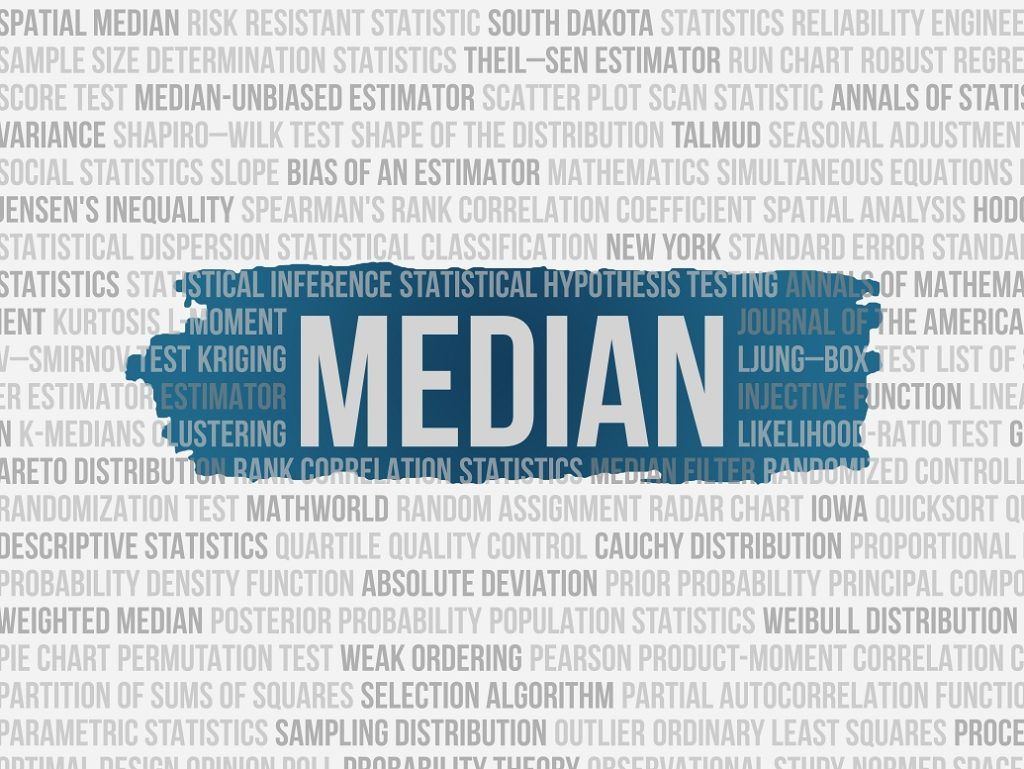Although mean is the most commonly used measure of central tendency for quantitative data, median can be used instead if the data contains large outliers. The outliers generally skew the mean while the median is not affected by extreme values. Sometimes the two measures are used simultaneously to determine the value that describes the central value the best.

### How to Find the Median?

The median can be easily found. In some cases, it does not require any calculations at all. The general steps of finding the median include:

1. Arrange the data in ascending order (from the lowest to the largest value).
2. Determine whether there is an even or an odd number of values in the dataset.
3. Considering the results of the previous step, further analysis may follow two distinct scenarios:
4. If the dataset contains an odd number of values, the median is a central value that will split the dataset into halves.
5. If the dataset contains the even number of values, find the two central values that split the dataset into halves. Then, calculate the mean of the two central values. The mean is the median of the dataset.

### Example of Median

You are an equity research analyst. Currently, you are working on the valuation of one of the large-cap IT companies in the market. You’ve already created a DCF model, but you want to check the results of the model using comparable analysis.

For the comparable analysis, you’ve determined the pool of companies. The primary analysis metric is the EV/EBITDA multiple. Since the obtained data contains some extreme values, you believe that the median will be the best central value in such a scenario.

Let’s consider the two scenarios:

Scenario 1: You identified five comparables.

The multiples of the comparable companies are summarized in the table below: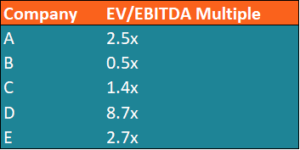In such a case, the median multiple can be found using the following steps:

1. Arrange the data in ascending order.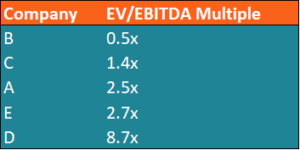1. The dataset contains an odd number of values. Thus, the median is the central value that splits the dataset into halves. In our example, it is the 5x multiple.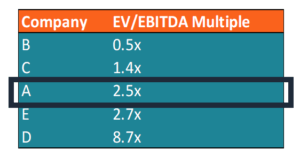Scenario 2: There are six comparable companies.

The table below summarizes the data for the second scenario: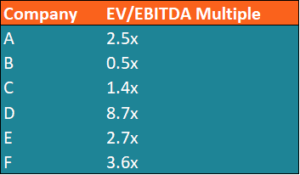The median for the dataset can be found in the following way:

1. Arrange the data in the ascending order.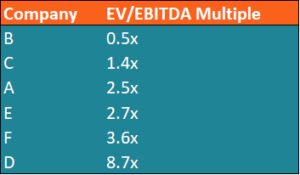1. The dataset contains an even number of values. Therefore, we need to find two central values that will split the dataset into halves.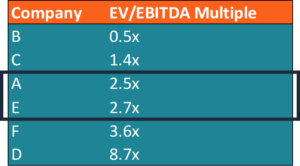1. The median of the dataset is the average of the two central values indicated above.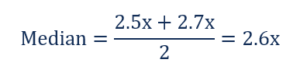### More Resources

CFI is the official provider of the global Financial Modeling & Valuation Analyst (FMVA)™ certification program, designed to help anyone become a world-class financial analyst. To keep advancing your career, the additional resources below will be useful:

• Basic Statistics Concepts in Finance
• DCF Model Template
• Excel Shortcuts for PC and Mac
• List of Excel Functions

### Financial Analyst Certification

Become a certified Financial Modeling and Valuation Analyst (FMVA)® by completing CFI’s online financial modeling classes and training program!